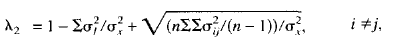# Guttman’s lambda-2: Definition, Examples

Statistics Definitions > Guttman’s lambda-2

## What is Guttman’s lambda-2?

Guttman’s lambda-2 (Guttman’s λ2) is a reliability estimate. λ-2 is the second in a series of 6 lambda’s proposed by Guttman in 1945. Lambda-1 was intended as a starting point for reliability analysis. From the 6 lambdas, lambda-2 and lambda-3 (which is equivalent to Cronbach’s alpha) are the most commonly used.

## Guttman’s lambda-2 vs. Alpha

Lambda-2 is similar to Cronbach’s alpha. While λ-2 is an estimate of between-score correlation for parallel measures, alpha is an estimate of between-score correlation for randomly equivalent measures. When you have composite tasks, λ-2 is thought to be more robust than alpha. Lambda-2 will always be greater than or equal to alpha for the test.

## Formula and Computation

The formula for Guttman’s lambda-2 is:Where σi,j is the covariance between items i and j.

You probably don’t want to calculate λ-2 by hand: the computations can get excessive, especially with larger tests. Many statistical packages can compute λ-2, including R And SPSS:

• In R, use the general command for reliability: check.reliability(X, MS = TRUE, alpha = TRUE, lambda.2 = TRUE, LCRC = FALSE, nclass = nclass.default). When lambda.2 = TRUE, it returns the λ-2 value.
• In SPSS, Choose Analyze > Scale > Reliability Analysis. Choose “Guttman” from the drop-down Model selection box.

## Interpretation of Guttman’s lambda-2

Reliability tests tell you how well test-takers can be differentiated by their scores. The lambda-2 statistic tells you what variance is due to true scores. If test-takers differ significantly in their abilities, λ-2 will be high and the error will be low. For example, a λ-2 of .79 means that 79% of the variance is due to true scores and 21% is due to error. The higher the λ-2, the better the test is able to differentiate between test-takers’ abilities.

Ideally, every test should be able to differentiate between test-takers’ abilities (otherwise there wouldn’t be any point in testing!). General rules of thumb for interpreting reliability estimates, including λ-2:

• ≥0.70 for group-level studies like evaluations.
• ≥0.80 for low-stakes evaluations such as instructional feedback or course grades.
• ≥0.90 for high-stakes decisions like school admissions, remedial placement, or promotions.

Notes:
What are Parallel measures?

Parallel measures are equivalent in content, response processes and statistical characteristics. For example, several tests exist for calculating IQ scores, and these are generally thought to be equivalent, or parallel.

What is Randomly equivalent?

Randomly equivalent tests are where test-takers take different tests. Differences in scores should be due to differences in test difficulty, as the underlying true score distributions are the same. This compares to non-equivalent tests where the test-takers come from different populations (i.e. the underlying true scores are not the same).

References:
Callender JC, Osburn HG. An empirical comparison of Coefficient Alpha, Guttman’s Lambda – 2, and MSPLIT maximized split-half reliability estimates. Journal of Educational Measurement. 1979;16(2):89–99.
Guttman, L. (1945). A basis for analyzing test-retest reliability. Psychometrika, 10, 255-282
Osburn, H.G. (2000). Coefficient Alpha and Related Internal Consistency Reliability Coefficients. Psychological Methods. Vol.5, No.2, 343-355. Retrieved 3/9/2017 from: https://pdfs.semanticscholar.org/c65a/b8997cb48561a76c922ec971eb434986e78c.pdf

CITE THIS AS:
Stephanie Glen. "Guttman’s lambda-2: Definition, Examples" From StatisticsHowTo.com: Elementary Statistics for the rest of us! https://www.statisticshowto.com/guttmans-lambda-2/